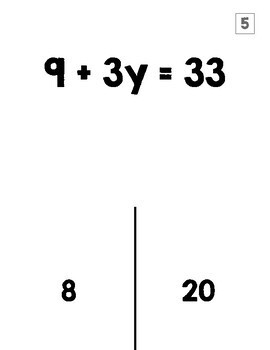# Two-Step Equations Halloween Activity TRICK or TREAT Game7th - 8th
Subjects
Standards
Resource Type
Formats Included
• PDF
Pages
20 pages

### Description

This fun Trick or Treat activity has students answer questions with 2 answer choices. One is a "trick" (incorrect answer) and one is a "treat" (correct answer). This Halloween game has students solve 16 two step equations and then lift a flap to see if they were tricked or not. Students love these... especially when real treats are involved! I hope you love it and your students learn it!

Need a digital version? Check out this Two-Step Equations Digital Trick or Treat Activity.

You may also like...

Two-Step Equations Candy Corn Worksheet

One-Step Equations TRICK or TREAT Halloween Activity

Multi-Step Equations Candy Corn Worksheet

Multi-Step Equations Halloween Color Page

Total Pages
20 pages
Included
Teaching Duration
30 minutes
Report this Resource to TpT
Reported resources will be reviewed by our team. Report this resource to let us know if this resource violates TpT’s content guidelines.

### Standards

to see state-specific standards (only available in the US).
Solve linear equations with rational number coefficients, including equations whose solutions require expanding expressions using the distributive property and collecting like terms.
Solve linear equations in one variable.
Solve word problems leading to equations of the form 𝘱𝘹 + 𝘲 = 𝘳 and 𝘱(𝘹 + 𝘲) = 𝘳, where 𝘱, 𝘲, and 𝘳 are specific rational numbers. Solve equations of these forms fluently. Compare an algebraic solution to an arithmetic solution, identifying the sequence of the operations used in each approach. For example, the perimeter of a rectangle is 54 cm. Its length is 6 cm. What is its width?